# Selecting External Components and Compensation for Automotive Step-Up DC-DC Regulator with Preboost Reference Design

### Abstract

In this application note, the parameters and calculations needed in the selection of external components for optimal performance of the MAX16990/MAX16992 in boost configurations are reviewed. Next, the selection of compensation components is discussed and a general method that can be extrapolated to compensate any boost regulator is offered. A calculator is provided to help the user in the selection of external components, compensation design, and the evaluation of power-supply performance. A reference design, showing how the devices can be used in an automotive preboost application, is discussed as is the optimal layout for this boost regulator.

### Introduction

A high-voltage boost controller, such as the MAX16990 or the MAX16992, the latter of which has 2.2MHz switching frequency capabilities, has many applications in the automotive field. Two uses are as a preboost regulator to sustain system voltage during cold-/warm-crank or as a power supply for high-brightness LEDs.
In this application note, we begin by examining how to realize an automotive high-voltage step-up DC-DC power supply with the MAX16990/MAX16992 and how to select the external components to achieve best system performance. Afterwards, we present a reference design for its preboost application.

### Parameters for Choosing External Components

There are four principal design input parameters for choosing external components for optimal performance of the MAX16990 and the MAX16992.
1. Switching frequency (fSW)
2. Output voltage (VOUT)
3. Output current range (IOUTMIN and IOUTMAX)
4. Input voltage range (VINMIN and VINMAX)
The MAX16990 and the MAX16992 operate in different switching frequency ranges, 100kHz to 1MHz for the former and 1MHz to 2.5MHz for the latter. Choose the version for the switching frequency you need.
Everything about the output stage (i.e., voltage and current range) is known. However, we only know the voltage range on the input stage. It would be useful to estimate the average input current range. We can do this with the following two equations:(Eq. 1)(Eq. 2)
Where the parameter Eff is the estimated efficiency of the boost regulator. We can extrapolate an initial estimation for Eff from the Typical Operating Characteristics in the MAX16990/MAX16992 data sheet and refine the estimation with the calculator after dimensioning all the external power components (nMOS, inductor, sense resistor, and rectifier diode).
Next, we need to evaluate the duty cycle range (DMIN and DMAX) where the regulator operates. This can be determined with the following two equations:(Eq. 3)(Eq. 4)
Where VD is the forward voltage of the rectifier diode, RDS(ON) the drain-source resistance of the nMOS when turned on, and RSENSE the sense resistor. Because we have not chosen RSENSE yet, ignore this term in the equations for now. We will make a more accurate estimate of the duty cycle range later.
Ensure that the estimated duty cycle range is within the specification of the selected device: 4% to 93% for the MAX16990 and 24% to 85% for the MAX16992.

### Inductor

To guarantee continuous-conduction mode (CCM) operation throughout the application, choose an inductor (L) higher than the critical inductance (LC) as calculated with Equation 5: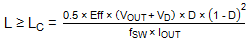(Eq. 5)
LC assumes its maximum value for D = 33% if it is in the calculated duty cycle range; otherwise choose the maximum value for LC between the ones calculated at the maximum and minimum duty cycles.
The other aspect to keep in mind when choosing the proper inductor is the LIR factor. This parameter is defined as the ratio of the peak-to-peak inductor current and the average input current:(Eq. 6)
The relationship between the inductor (L) and the LIR factor is shown in Equation 7: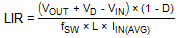(Eq. 7)
To reduce losses, choose an inductor that guarantees an LIR factor between 0.3 and 0.5. With L equal to LC, the LIR factor is 2. Further increasing L reduces the LIR factor. The selected inductor has to have a saturation current higher than its peak current, which is:(Eq. 8)
Figure 1 illustrates the inductor current shape during the switching period.Figure 1. Inductor current of the boost regulator.
The peak inductor current coincides with the peak nMOS current and rectifier diode current. Considering this, choose the current rating of the two power components accordingly. Additionally, the maximum nMOS drain-source voltage is equal to the output voltage (VOUT) plus the drop on the rectifier diode (VD), and the maximum reverse voltage across the rectifier diode is equal to the output voltage (VOUT).

### Sense Resistor

Now that the peak inductor current has been calculated, it is possible to select the sense resistor (RSENSE). The device triggers the current limit when the voltage on the ISNS pin reaches 212mV (min). A portion of this voltage is due to the drop on the sense resistor and another portion to the drop on the slope resistor (RSLOPE), which is used for slope compensation. To leave 100mV of room for slope compensation, it is initially recommended for RSENSE to generate a voltage drop of 112mV at the current limit threshold. In Equation 9, RSENSE is calculated with a current limit threshold 20% higher than the peak inductor current.(Eq. 9)

### Output Capacitor

Selecting the correct output capacitor (COUT) and its related ESR is very important to minimize output voltage ripple.
Assume that the output voltage ripple (VOUT_RIPPLE) is equally distributed between the voltage drop, which is due to the capacitor discharging during off-time, and the ESR voltage drop.(Eq. 10)(Eq. 11)

### Compensation

After looking at these external components (the inductor, sense resistor, and output capacitor), we need to consider the external compensation components necessary for the preboost regulator. See Figure 2 for an overview of the boost regulation loop, which is composed of the power stage (A(f)) and the feedback stage (B(f)).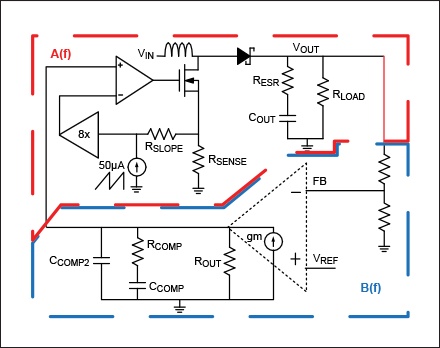Figure 2. Boost regulator small-signal model.
In order to select the appropriate external compensation components (RCOMP, CCOMP, CCOMP2, and RSLOPE), it is necessary to describe the loop response in the frequency domain and evaluate its stability. The regulation loop can be divided into two stages.
The first stage, A(f), is the power stage, which is composed of the current-sense circuitry, the PWM comparator, the external nMOS, the inductor (L), the output capacitor (COUT), and the load resistor (RLOAD). The frequency response of this stage is described by Equation 12:(Eq. 12)
The DC gain ACM is: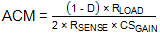(Eq. 13)
The numerator in Equation 12 is composed of the zero introduced by the output capacitor ESR:(Eq. 14)
And the right-half plane zero of the current-mode boost regulator:(Eq. 15)
It is useful to remember that this zero acts as a normal zero from the module side but as a pole from the phase side, thereby decreasing the phase of the closed-loop frequency response.
The A(f) denominator in Equation 12 is composed of the output pole: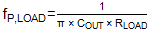(Eq. 16)
And the double pole at half of the switching frequency, which has to be damped with slope compensation.
The second stage that characterizes the closed-loop response, B(f), is calculated with the feedback network (AFB) and the error amplifier (AEA):(Eq. 17)
The DC gain is calculated from the AFB and AEA gains:
 AFB = VREF/VOUT (Eq. 18) AEA = gm × ROUT (Eq. 19)
Where gm is the voltage-to-current gain of the transconductance error amplifier and ROUT its output.
The error amplifier zero and main pole are determined by the external compensation components CCOMP and RCOMP:(Eq. 20)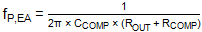(Eq. 21)
A second error amplifier pole can be added, if needed, with a capacitor between the COMP pin and GND (CCOMP2):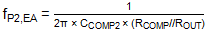(Eq. 22)
The closed-loop response of the regulator is achieved by tying together A(f) and B(f):
 Loop(f) = A(f) × B(f) (Eq. 23)
Once we become familiar with the loop frequency response, the first step to ensure stability is to select the proper slope compensation in order to avoid oscillation at half of the switching frequency. To do that, the Q factor, shown in Equation 24, has to be between zero and one:(Eq. 24)
Where Sn is the positive inductor current ramp during on-time multiplied by the sense resistor (voltage ramp on RSENSE):(Eq. 25)
And Se is the slope compensation ramp multiplied by RSENSE plus RSLOPE:
 Se = ICOMP × fSW × (RSLOPE + RSENSE), ICOMP = 50µA (Eq. 26)
RSLOPE must have a Q factor between zero and one in all operating conditions.
The worst-case scenario for slope compensation is when the input voltage is at its minimum and the output current at its maximum.
Choosing a RSLOPE higher than the value shown in Equation 27 ensures a Q factor between 0 and 1 in all operating conditions:(Eq. 27)
Once RSLOPE has been selected, it is possible to calculate the value of the real minimum current limit using Equation 28:(Eq. 28)
If the current limit is too high, increase RSENSE and RSLOPE accordingly until the desired value is reached.
Ensure that the minimum current limit is higher than the peak inductor current.
Once the double pole at half of the switching frequency is dumped, it is necessary to choose the error amplifier compensation components to ensure good phase margin at the crossover frequency.
The first step is to choose the desired crossover frequency (fC,TARGET), which has to be lower than fSW/10 and fZ,RHP/10. Initially, we assume that the zero due to the output capacitor ESR (fZ,ESR) is ten times higher than fC,TARGET. Under this assumption, the closed-loop frequency response can be approximated as a simple two poles and one zero system frequency response.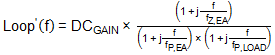(Eq. 29) DCGAIN = ACM × AFB × AEA (Eq. 30)
Based on the target crossover frequency and the obtained DCGAIN, two cases can be considered.
The first one is when: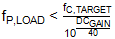(Eq. 31)
In this case (see Figure 3), place the error amplifier pole after the load pole :(Eq. 32)
And the error amplifier zero exactly on the target crossover frequency:(Eq. 33)
This ensures a 45° positive lag on the phase margin.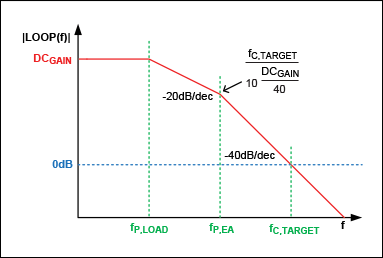Figure 3. Bode diagram of the amplitude of the closed-loop response, case 1.
The second one is when:(Eq. 34)
In this case (see Figure 4), place the error amplifier pole before the load pole:(Eq. 35)
And the error amplifier zero exactly on the target crossover frequency:(Eq. 36)
This ensures a 45° positive lag on the phase margin.Figure 4. Bode diagram of the amplitude of the closed-loop response, case 2.
Use the calculator to estimate the obtained crossover frequency and phase margin. If they are not satisfactory, increase RCOMP to increase the crossover frequency and the phase margin.
If the zero from the output capacitor ESR is not negligible and affects the phase margin and crossover frequency, add a second error amplifier pole (CCOMP2) corresponding to the ESR zero:(Eq. 37)

### Reference Design

After discussing the external and compensation components required, we consider a reference design for an automotive preboost application.
The usual requirements for an automotive preboost application are:
 fSW 2.2MHz VIN 3.5V to 6V VOUT 8V IOUT 1A to 2A VOUT_RIPPLE 50mV
Estimating an efficiency (Eff) of 90%, the input current range should be:(Eq. 38)(Eq. 39)
The second step is to calculate the duty cycle range. To do that, it is useful to choose the nMOS resistor. In order to determine the nMOS ratings requirement, it is necessary to calculate the peak transistor current (corresponding to the peak inductor current).
Assume a maximum LIR of 0.5 when the input current is at its maximum:(Eq. 40)
Based on this information, Fairchild’s FDS5670 nMOS, which is rated for a drain current of 10A, was chosen. The typical RDS(ON) of this transistor is 15mΩ with a VGS = 5V (the gate-source voltage of the MAX16992).
Once we have this information, we can calculate the duty cycle range ignoring RSENSE for now:(Eq. 41)(Eq. 42)
Assume that the forward voltage of the rectifier diode (Diodes Incorporated’s B3x0-13-F) is equal to 0.5V. The duty cycle range is compatible with the MAX16992. To guarantee continuous-conduction mode:(Eq. 43)
In the worst-case scenario, D = 0.33% and IOUT = 1A.
Based on this information, Würth Elektronik’s 0.47µH inductor 744314047 was selected (IR = 18A, ISAT = 20A). With this inductor, when the input voltage is at its minimum (and the input current at its maximum):(Eq. 44)
Resulting in an inductor (and nMOS) peak current of:(Eq. 45)
This value is in accordance with the nMOS drain current rating.
Now it is possible to calculate the sense resistor:(Eq. 46)
A 15mΩ resistor was chosen for RSENSE.
In accordance with the design specification on the output voltage ripple, the constraints on COUT are:(Eq. 47)(Eq. 48)
Murata’s 47µF GRM32ER61C476K capacitor with an ESR of 3mΩ at the switching frequency 2.2MHz was chosen.
The first parameter to select for compensation is RSLOPE: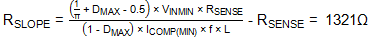(Eq. 49)
A standard 1.3kΩ resistor was chosen. The minimum current limit threshold became: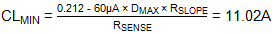(Eq. 50)
The DCGAIN, load pole frequency, and the right-half plane zero frequency are:
 DCGAIN = ACM × AFB × AEA = 91.6dB (Eq. 51)(Eq. 52)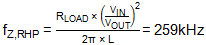(Eq. 53)
Which are calculated for the worst-case scenario with the input voltage at its minimum and the load current at its maximum.
Murata’s 47µF capacitor has an ESR lower than 20mΩ for a frequency above 2kHz.
Thus, the ESR zero, in the worst case, is:(Eq. 54)
In this case, the maximum crossover frequency has to be lower than fZ,RHP/10 = 25.9kHz.
Choosing a target crossover frequency of 25kHz, we must follow: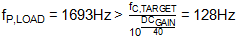(Eq. 55)
In this case, the CCOMP target becomes:(Eq. 56)
A standard 470pF capacitor was chosen and consequently the estimated RCOMP target is:(Eq. 57)
A standard 15kΩ resistor was chosen.
The last component remaining is CCOMP2:(Eq. 58)
A standard 68pF capacitor was chosen.
With the chosen external compensation components, the error amplifier zero and pole frequencies are:(Eq. 59)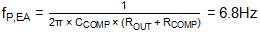(Eq. 60)(Eq. 61)
Use the calculator to determine the obtained crossover frequency (fCROSS) and phase margin (PM).
In this case, these two parameters are:
 fCROSS = 26.3kHz (Eq. 62) PM = 45° (Eq. 63)
The final Bode diagrams of the closed loop regulator are illustrated in Figure 5 and Figure 6.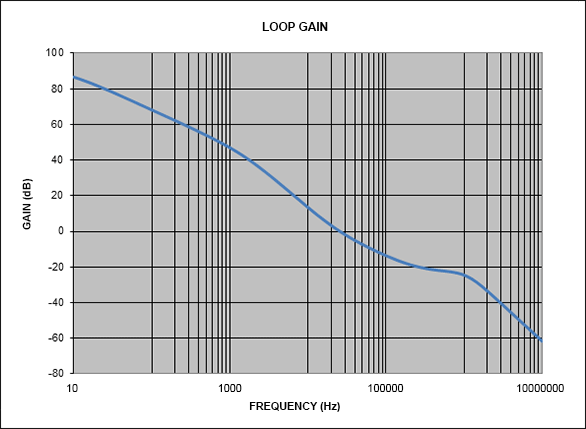Figure 5. Loop gain.Figure 6. Loop phase.
 Designation Description N Fairchild FDS5670 nMOS D Diodes Inc. B3x0-13-F L Würth Elektronik 744314047 COUT Murata GRM32ER61C476KFigure 7. Schematic of reference design.

### Layout Recommendation

A good layout is very important to maximize EMI and jitter-free performance of the boost regulator. To achieve that, follow these general recommendations:
1. Place all power components on the same side of the board.
2. Keep the AC paths as short as possible. During on-time, the AC path is composed of CIN, an inductor, nMOS, RSENSE, and GND. During off-time, the AC path is composed of CIN, an inductor, a diode, COUT, and GND.
3. Keep the switching node (LX) as compact as possible.
4. Do not route the path between the DRV pin and the gate of the nMOS with the minimum width. This net commutes at the switching frequency and has to carry the current necessary to drive the nMOS. If vias are necessary, route the net to an internal layer.
5. Connect the CSUP and CPVL capacitors directly to the IC, as close as possible without using vias.
6. Use a Kelvin connection between RSENSE and RSLOPE, and between RSLOPE and the ISNS pin.
7. Use a Kelvin connection between OUT and RTOP. Keep the FB node as close as possible to the FB pin of the IC.
8. Use two separate GNDs as indicated on the schematic: PGND for power components and AGND for the signal circuitry and the EP of the MAX16992. Use a single-point connection between PGND and AGND, as close as possible to the EP.
A reference layout is shown in Figure 8 through Figure 12.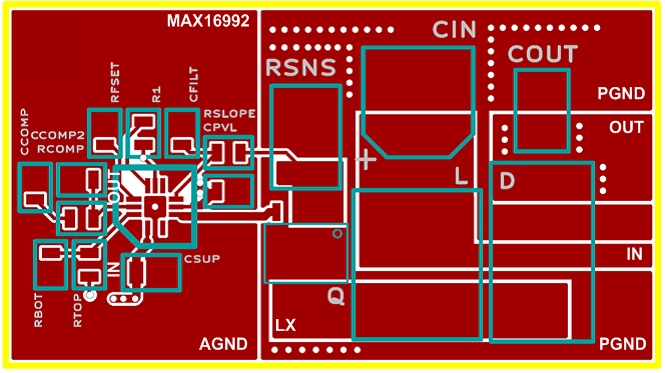Figure 8. Reference design layout, top layer.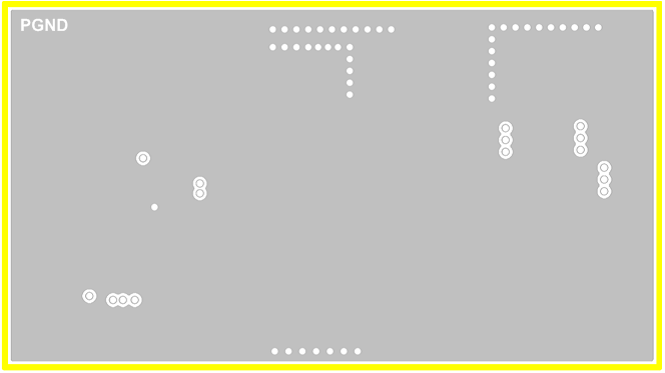Figure 9. Reference design layout, inner layer 1.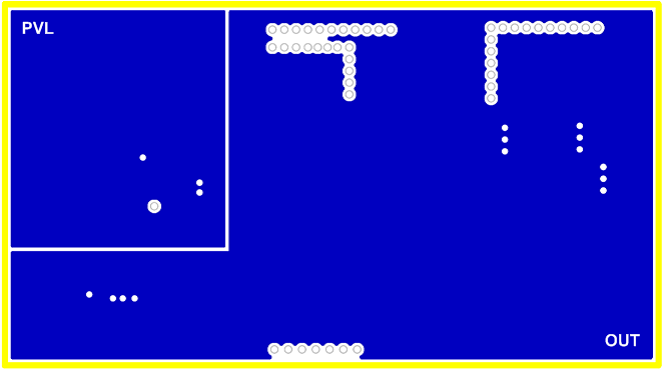Figure 10. Reference design layout, inner layer 2.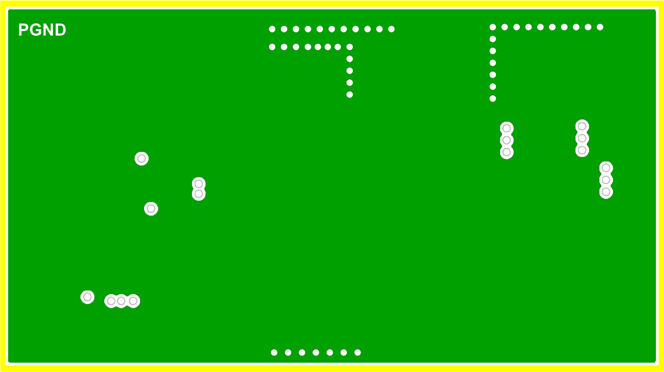Figure 11. Reference design layout, back layer.Figure 12. Reference design, 3D view.

### Conclusion

In this application note, we learned how best to select external components and compensation for optimal performance of the MAX16990/MAX16922. We then saw how these devices can be used in automotive applications as preboost regulators and discovered the best layout to maximize EMI and minimize jitter.